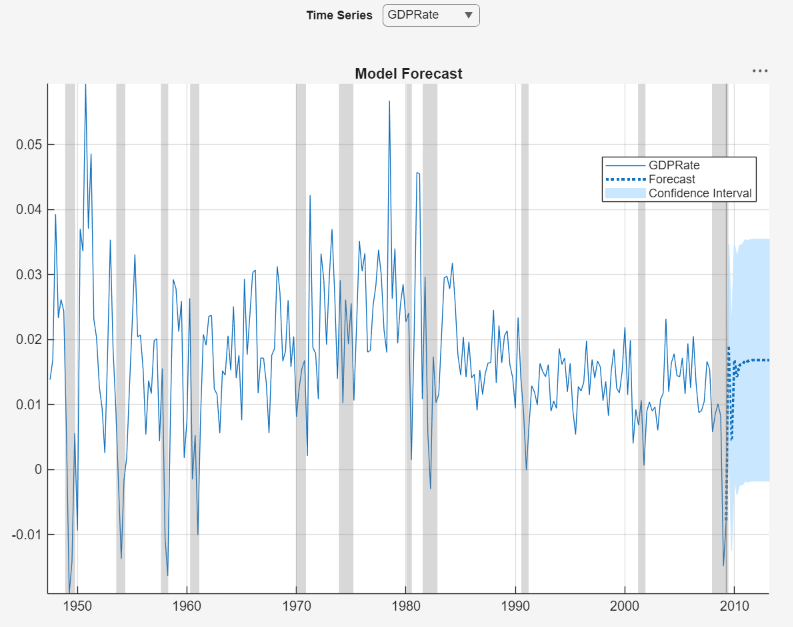## Estimate Vector Autoregression Model Using Econometric Modeler App

This example models the quarterly US GDP growth rate, M1 money supply rate, and the 3-month T-bill rate series by using the Econometric Modeler app. The example performs the following actions in the app:

1. Stabilize the raw nonstationary series.

2. Fit several completing vector autoregression (VAR) models, and choose the one with the best, parsimonious fit.

3. Diagnose each residual series.

4. Export the chosen model to the command line.

At the command line, the example conducts Granger-causality tests on the series given the estimated model, and it uses the model to generate forecasts.

The data set, which is stored in `Data_USEconModel.mat`, contains the raw, quarterly US GDP, M1 money supply, and 3-month T-bill rate, among other series, from 1947 through 2009.

### Load and Import Data into Econometric Modeler

At the command line, load the `Data_USEconModel.mat` data set.

`load Data_USEconModel`

At the command line, open the Econometric Modeler app.

`econometricModeler`

Alternatively, open the app from the apps gallery (see Econometric Modeler).

Import `DataTable` into the app:

1. On the Econometric Modeler tab, in the Import section, click.

2. In the Import Data dialog box, in the Import? column, select the check box for the `DataTable` variable.

3. Click .

`GDP`, `M1SL`, and `TB3MS`, among other series, appear in the Time Series pane, and a time series plot containing all series appears in the figure window.

Create separate time series plots by double-clicking each of `GDP`, `M1SL`, and `TB3MS` in the Time Series pane. Position the Time Series Plot(`series`) tabs by clicking and dragging each to see all plots simultaneously.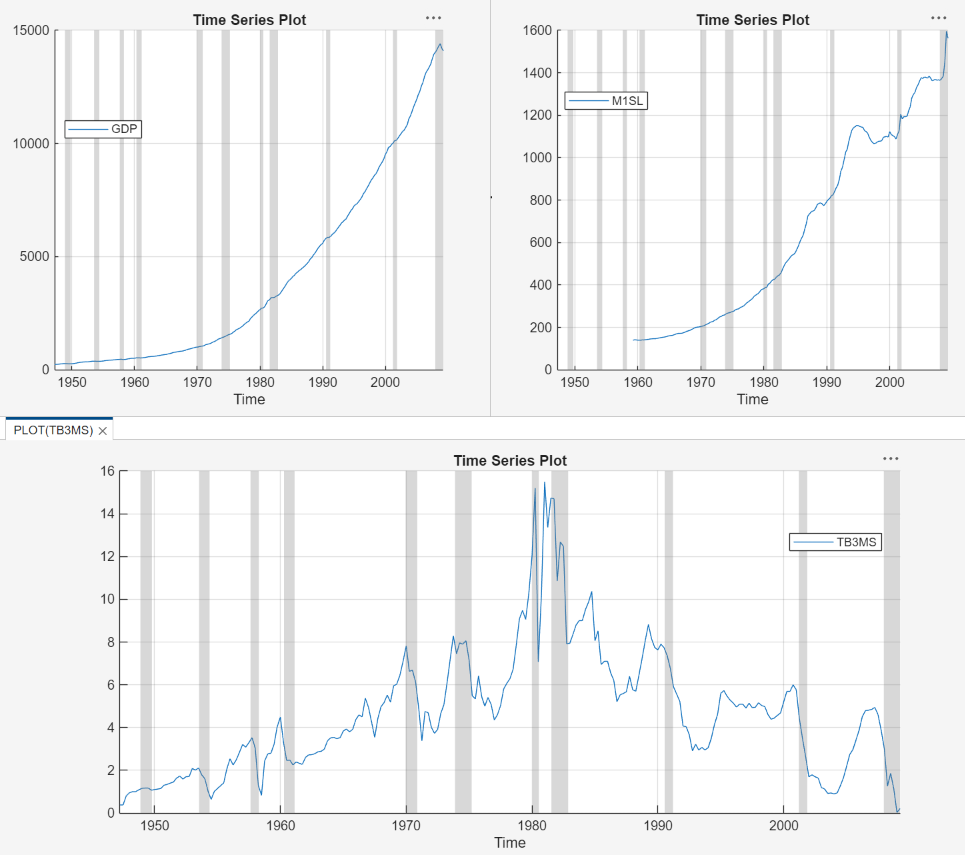The US GDP and M1 money supply series exhibit exponential growth, and the 3-month T-Bill series appears like a random walk.

### Diagnose and Transform Series

Remove the exponential trend from the GDP and M1 money supply series by applying the log transform to each series. Click the Econometric Modeler tab, and then, in the Time Series pane, click `GDP` and Ctrl click `M1SL`. In the Transforms section, click . The transformed series `GDPLog` and `M1SLLog` appear in the Time Series pane, and their time series plot appears in the Time Series Plot(GDPLog) figure window.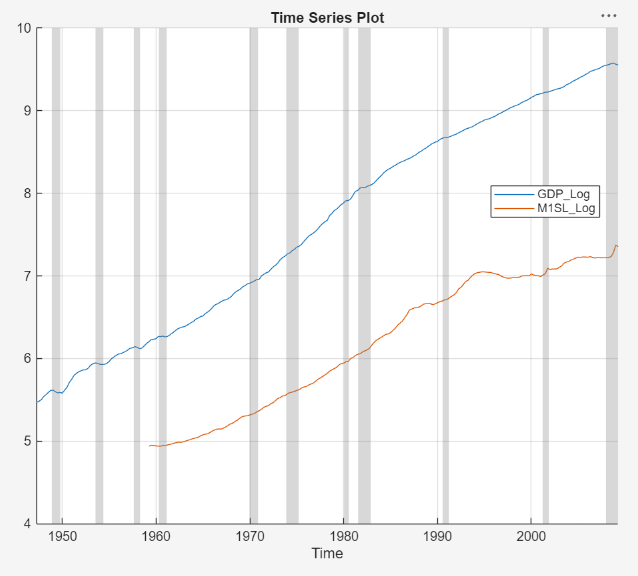Test the null hypothesis that each series is a unit root process against a stationary AR(`p`) with drift alternative, where `p` = 4 through 1. For each series `GDPLog`, `M1SL`, and `TB3MS`:

1. On the Econometric Modeler tab, in the Time Series pane, click the series.

2. On the Econometric Modeler tab, in the Time Series section, click > .

3. On the ADF tab, in the Parameters section, in the Number of Lags box, type `4`.

4. In the Tests section, Click .

5. Repeat steps 3 and 4 for lags `3`, `2`, and `1`.

The following figures show the results. The tests fail to reject the null hypotheses of a unit root series in all cases, which suggests that each series is difference stationary.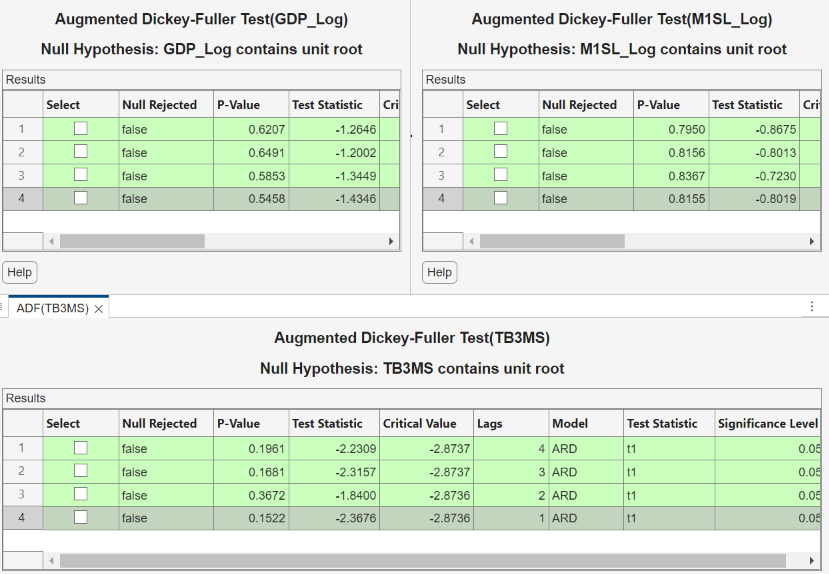Stabilize the series by applying the first difference to each series.

1. Click the Econometric Modeler tab, and then, in the Time Series pane, click `GDPLog` and Ctrl click `M1SLLog` and `TB3MS`.

2. In the Transforms section, click . The transformed series `GDPLogDiff`, `M1SLLogDiff`, and `TB3MSDiff` appear in the Time Series pane, and their time series plot appears in the Time Series Plot(GDPLogDiff) figure window.

3. Rename `GDPLogDiff` and `M1SLLogDiff` to `GDPRate` and `M1SLRate` by clicking on their names twice in the Time Series pane, and typing their new names.

### Estimate VAR Models

Estimate 3-D VAR(`p`) models of the US quarterly GDP growth rate series `GDPRate`, M1 money supply growth rate series `M1SLRate`, and change in the 3-month treasury bill rate series `TB3MSDiff`, where `p` = 1 through 4.

1. In the Time Series pane, click `GDPLog` and Ctrl click `M1SLLog` and `TB3MS`.

2. In the Models section, click VAR.

3. Fit a VAR(1) model (the default) by clicking in the VAR Model Parameters dialog box.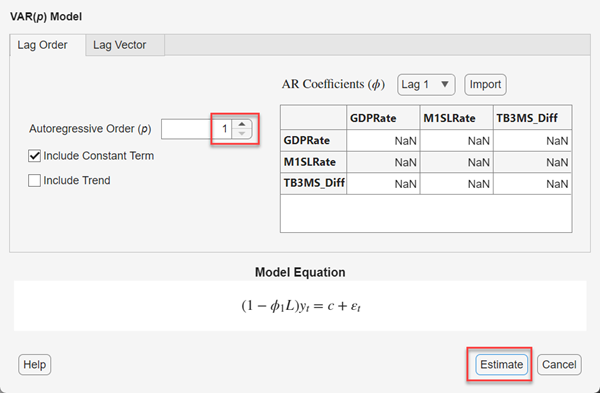The model variable `VAR` appears in the Models pane, its value appears in the Preview pane, and its estimation summary appears in the Model Summary(VAR) document.

4. Repeat steps 1 and 2 for each AR order `p` = 2 through 4. In the Var Model Parameters dialog, set the AR order by using the Autoregressive Order box.

Similar to the VAR(1) estimation, a variable for each model (`VAR2`, `VAR3`, and `VAR4`) appears in the appears in the Models pane, and their estimation summaries appear in their respective Model Summary(`ModelName`) document. You can view properties of an estimated model in the Preview pane by clicking the model in the Models pane. For example, click `VAR4`.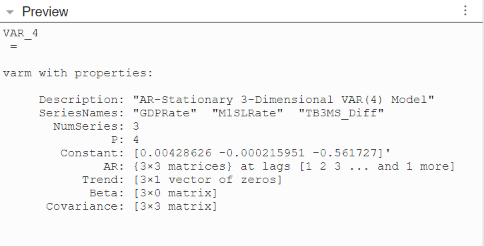### Select Model With Best In-Sample Fit

The estimation summary in each Model Summary(VAR`p`) tab contains a plot of fitted values and residuals, with respect to the time series in the Time Series list, a standard statistical table of estimates and inferences, and a table of information criteria.

Compare the information criteria of each estimated model simultaneously by positioning the estimation summary documents so that they occupy the four quadrants of the right pane. The model with the lowest value has the best, parsimonious fit.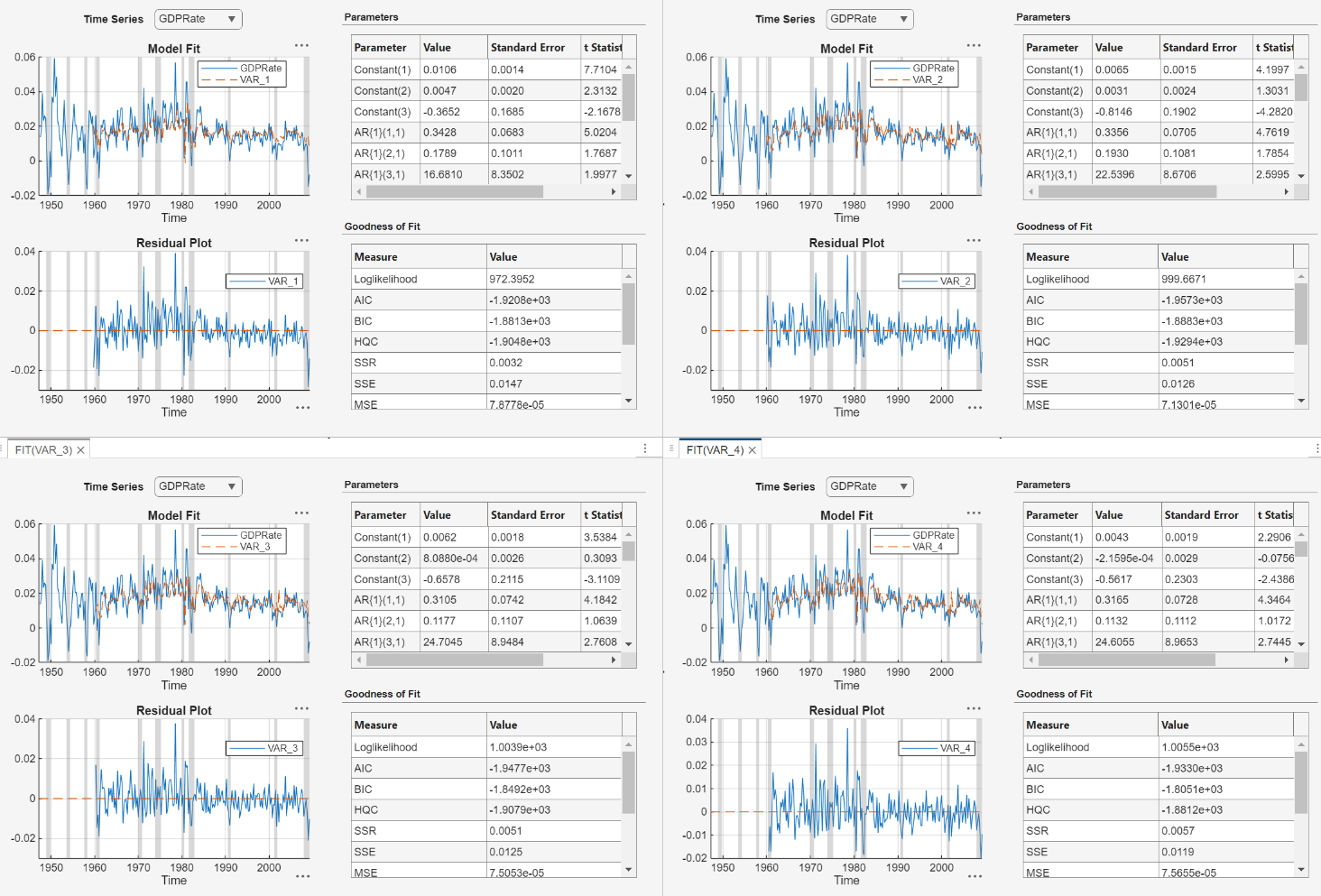The VAR(2) model `VAR2` produces the lowest AIC and BIC values. Choose this model for further analysis.

### Check Goodness of Fit

Inspect the following VAR(2) plots of each residual series:

• Histograms, for center, normality, and outliers

• Quantile-quantile plots, for normality, skewness, and tails

• Autocorrelation function (ACF), for serial correlation

• ACF of squared residual series, for heteroscedasticity

This example diagnoses the residuals visually. Alternatively, you can conduct statistical tests to diagnose the residuals.

In the Model Summary(VAR2) document, click > Tile All, and then click the Single radio button.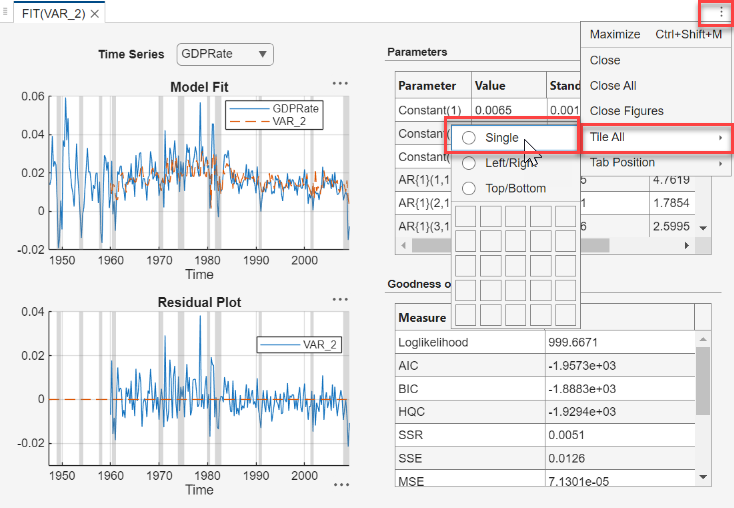On the Models pane, click `VAR2`.

Plot separate residual histograms. On the Econometric Modeler tab, in the Diagnostics section, click > Residual Histogram. Histograms of the each residual series appear in the Histogram(VAR2) document.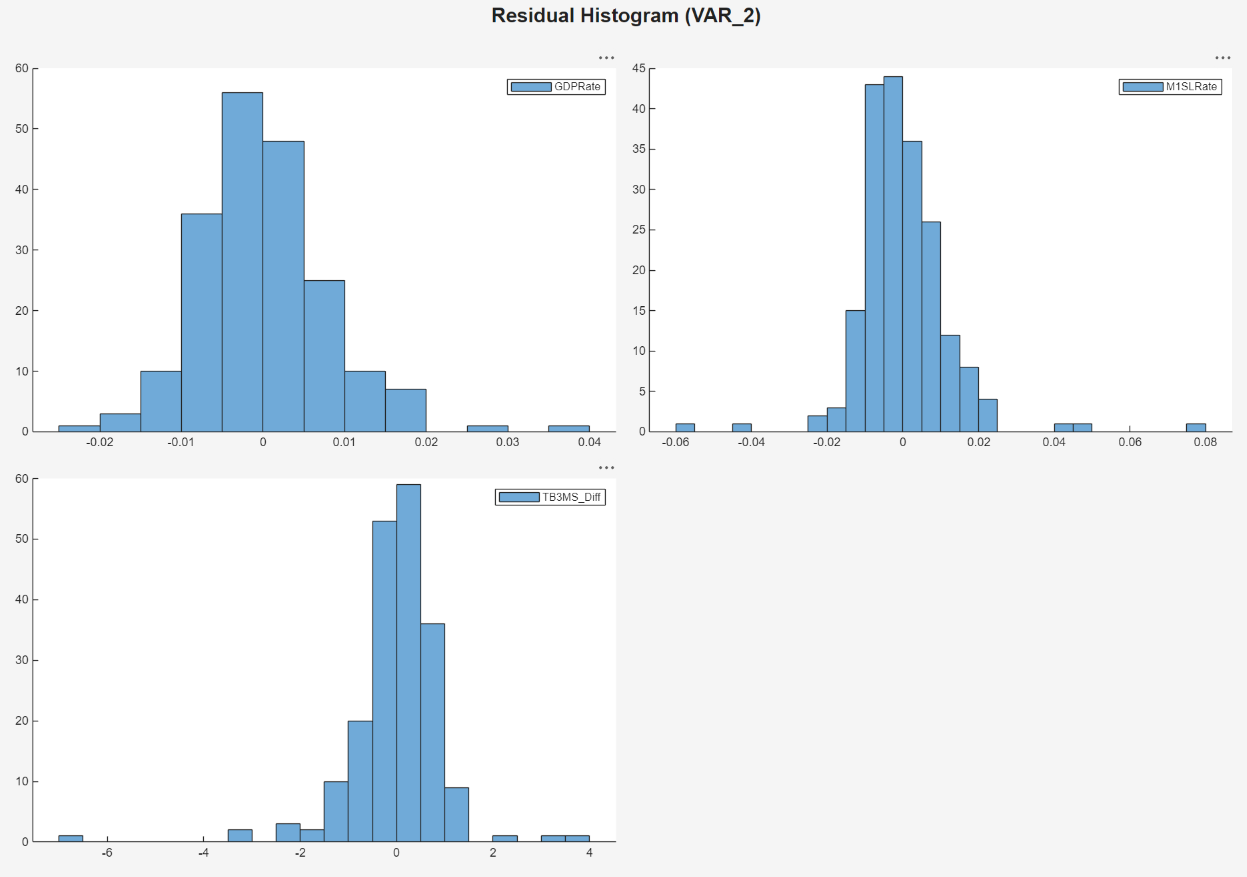Each residual series appears centered around 0, with varying degrees of slight skewness and possible outliers. This example proceeds without addressing possible skewness and outliers.

Plot separate residual quantile-quantile plots. With `VAR2` selected in the Time Series pane, on the Econometric Modeler tab, in the Diagnostics section, click > Residual Q-Q Plot. Quantile-quantile plots of the each residual series appear in the QQPlot(VAR2) document.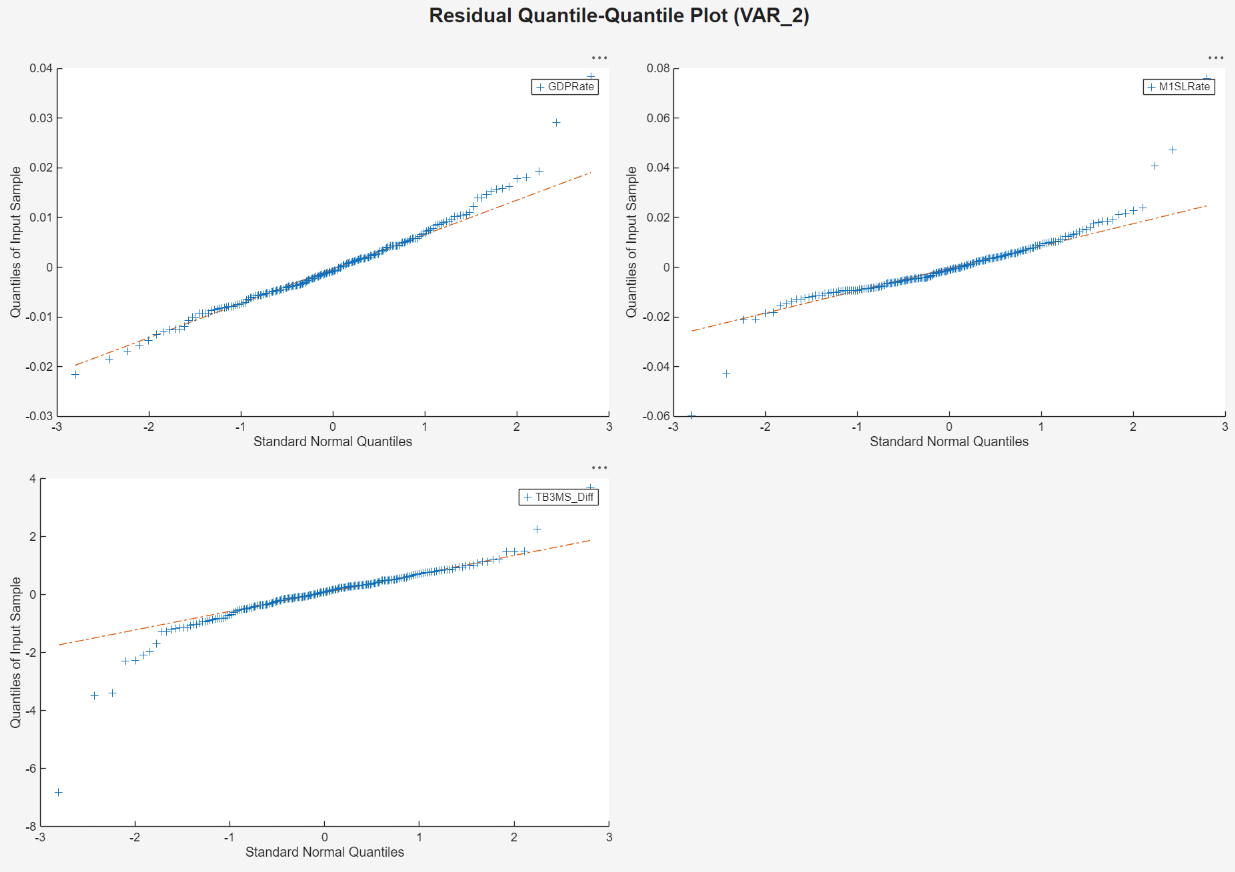Each series has slightly fatter tails than what is expected by the normal distribution. This example proceeds without addressing possible kurtosis.

Plot separate ACF plots of each residual series. With `VAR2` selected in the Time Series pane, on the Econometric Modeler tab, in the Diagnostics section, click > Autocorrelation Function. ACF plots of the each residual series appear in the ACF(VAR2) document.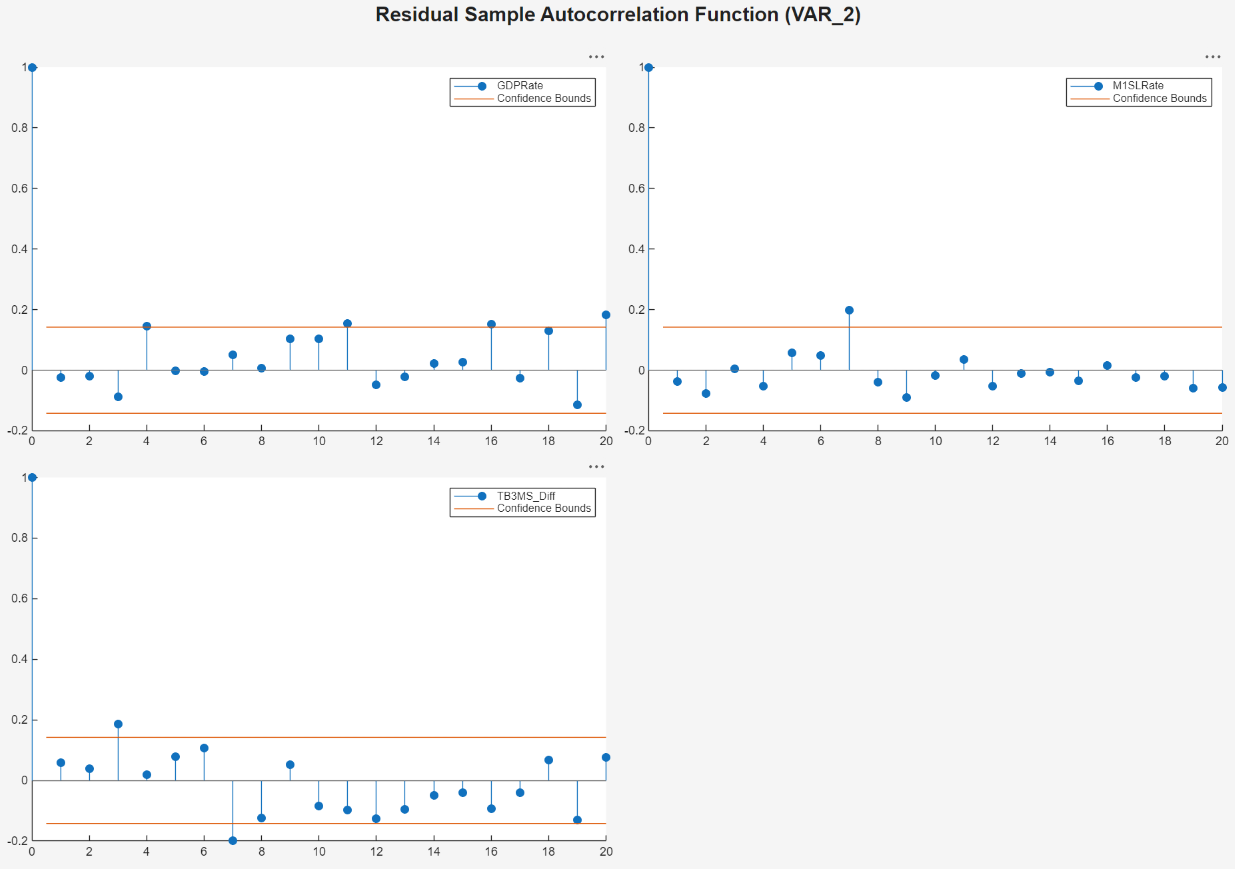Each series has several significant, albeit small, autocorrelations. For example, the `GDPRate` residual series has significant autocorrelations at lags 4 and 16, and the `M1SLRate` and `TB3MSDiff` quarterly difference series both have significant autocorrelations at lags 7. To address the autocorrelations, you can include higher AR lags in the VAR model for estimation, but this example proceeds without addressing the autocorrelations this way.

Plot separate ACF plots of each squared residual series. With `VAR2` selected in the Time Series pane, on the Econometric Modeler tab, in the Diagnostics section, click > Squared Residual Autocorrelation. ACF plots of the each squared residual series appear in the ACF(VAR2)2 document.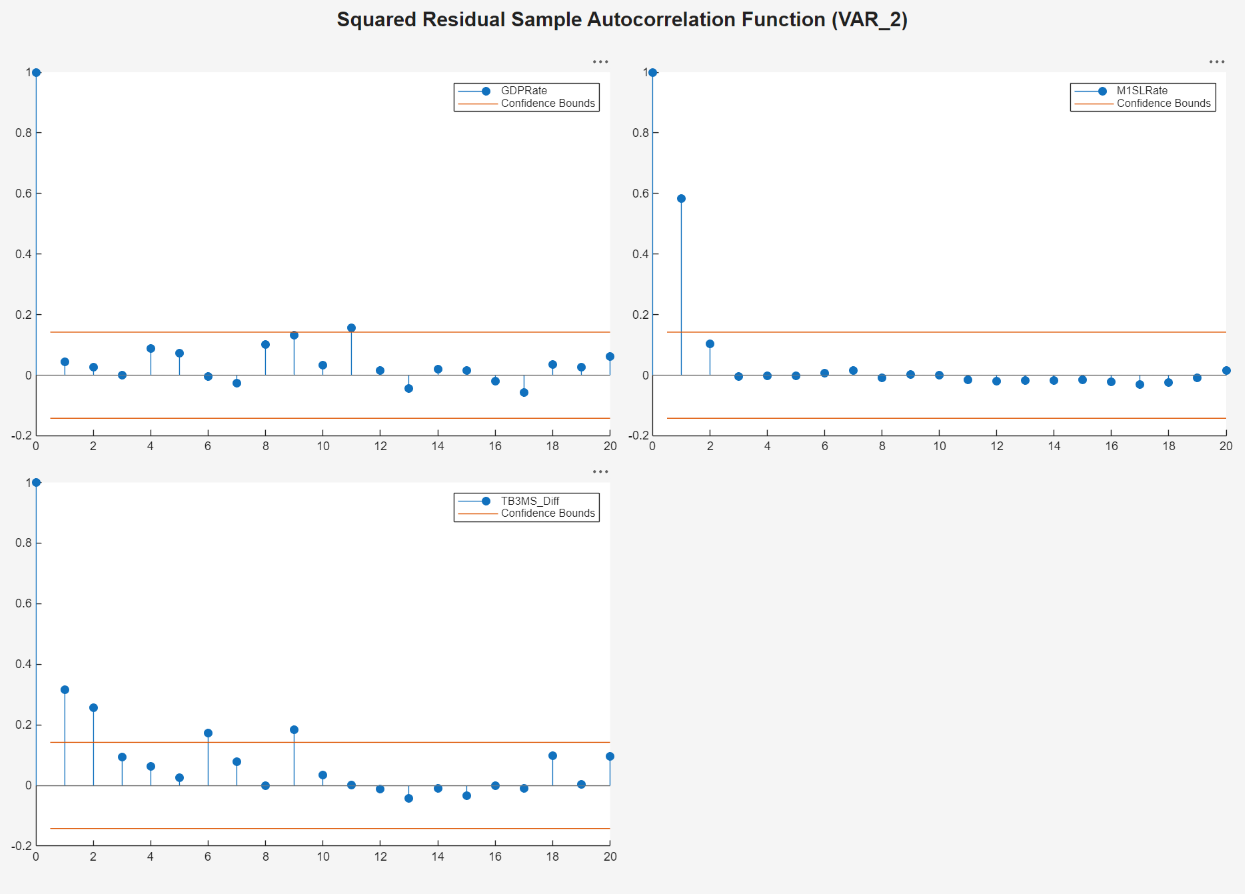The `M1SLRate` and `TB3MSDiff` series have significant autocorrelations, which suggests that heteroscedasticity is present. This example proceeds without addressing possible heteroscedasticity.

### Export Model to Workspace

Export the model to the workspace.

1. With the `VAR2` model selected in the Models pane, on the Econometric Modeler tab, in the Export section, click > .

2. In the Export Variables dialog box, select the Select check box the `GDPRate`, `M1SLRate`, and `TB3MSDiff`.

3. Click .

The variables `GDPRate`, `M1SLRate`, `TB3MSDiff`, and `VAR2` appear in the workspace.

### Conduct Causality Analysis at Command Line

Determine whether series in the system Granger-cause the other series by conducting 1-step, leave-one-out Granger-causality tests. Pass the estimated VAR(2) model to `gctest`.

`gctest(VAR2)`
``` H0 Decision Distribution Statistic PValue CriticalValue _______________________________________________ __________________ ____________ _________ __________ _____________ "Exclude lagged M1SLRate in GDPRate equation" "Reject H0" "Chi2(2)" 8.3934 0.015045 5.9915 "Exclude lagged TB3MSDiff in GDPRate equation" "Reject H0" "Chi2(2)" 16.924 0.00021133 5.9915 "Exclude lagged GDPRate in M1SLRate equation" "Cannot reject H0" "Chi2(2)" 3.1528 0.20672 5.9915 "Exclude lagged TB3MSDiff in M1SLRate equation" "Reject H0" "Chi2(2)" 20.855 2.9608e-05 5.9915 "Exclude lagged GDPRate in TB3MSDiff equation" "Reject H0" "Chi2(2)" 20.565 3.4229e-05 5.9915 "Exclude lagged M1SLRate in TB3MSDiff equation" "Cannot reject H0" "Chi2(2)" 0.41428 0.81291 5.9915 ```

The null hypothesis of the 1-step leave-one-out Granger-causality test is that a series does not 1-step Granger-cause another series, conditioned on all other series being present in the system. The results suggest:

• Given that the 3-month T-bill change is in the system, enough evidence exists to suggest that M1 money supply rate 1-step Granger-causes the GDP rate.

• Given that the M1 money supply rate is in the system, enough evidence exists to suggest that the 3-month T-bill change 1-step Granger-causes the GDP rate.

• Given that the 3-month T-bill change is in the system, not enough evidence exists to suggest that the GDP rate 1-step Granger-causes the M1 money supply rate.

• Given that the GDP rate is in the system, enough evidence exists to suggest that the 3-month T-bill change 1-step Granger-causes the M1 money supply rate.

• Given that the M1 money supply rate is in the system, enough evidence exists to suggest that the GDP rate 1-step Granger-causes the 3-month T-bill change.

• Given that the GDP rate is in the system, not enough evidence exists to suggest that the 1-step M1 money supply rate Granger-causes the 3-month T-bill change.

### Generate Forecasts at Command Line

Generate forecasts and approximate 95% forecast intervals from the estimated VAR(2) model for the next four years (16 quarters). For convenience, use the entire series as a presample for the forecasts (`forecast` discards all specified presample observations except for the required final two observations).

```Y = [GDPRate M1SLRate TB3MSDiff]; [YF,YFMSE] = forecast(VAR2,16,Y); YFSE = cell2mat(cellfun(@(x)sqrt(diag(x)'),YFMSE,UniformOutput=false)); UB = YF + 1.96*YFSE; LB = YF - 1.96*YFSE; datesF = DataTable.Time(end) + calquarters(1:16); figure tiledlayout(3,1) for j = 1:VAR2.NumSeries nexttile h1 = plot(DataTable.Time(end-30:end),Y0(end-30:end,j),Color=[.75,.75,.75]); hold on h2 = plot(datesF,YF(:,j),"r",LineWidth=2); h3 = plot(datesF,UB(:,j),"k--",LineWidth=1.5); plot(datesF,LB(:,j),"k--",LineWidth=1.5); ct = [Y0(end,j) YF(1,j); Y0(end,j) LB(1,j); Y0(end,j) UB(1,j);]; plot([DataTable.Time(end); datesF(1)],ct,Color=[.75,.75,.75]) legend([h1 h2 h3],VAR2.SeriesNames(j),"Forecast", ... "Forecast Interval",Location="northwest") hold off end```